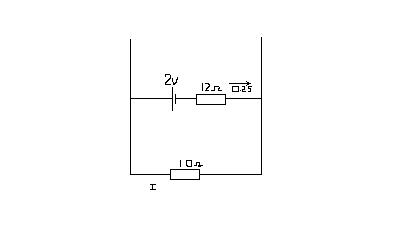# DC Circuit. Kirchoff's 2nd law.

Gregg

## Homework Statement

Is it possible to find the P.D. across the 10ohm resistor.## Homework Equations

Kirchoff's second law.

## The Attempt at a Solution

Well KSL states that the sum of the pds is equal to the sum of the emfs.

$I(10) + 0.25(12) = 2$

Gives the wrong value for I. I is 0.5. I took this part of the circuit out. Actually there is a source of emf in parallel with 5V flowing in the opposite direction to the 2V cell. I'm asking if it's possible to work out the pd across the 10ohm resister without that part of it, using Kirchoffs law for pds and emf in a closed loop.

EDIT: Oops.

$I(10) - 0.25(12) = 2$

$I = 0.5$

Sorry.

Why is the value of I wrong